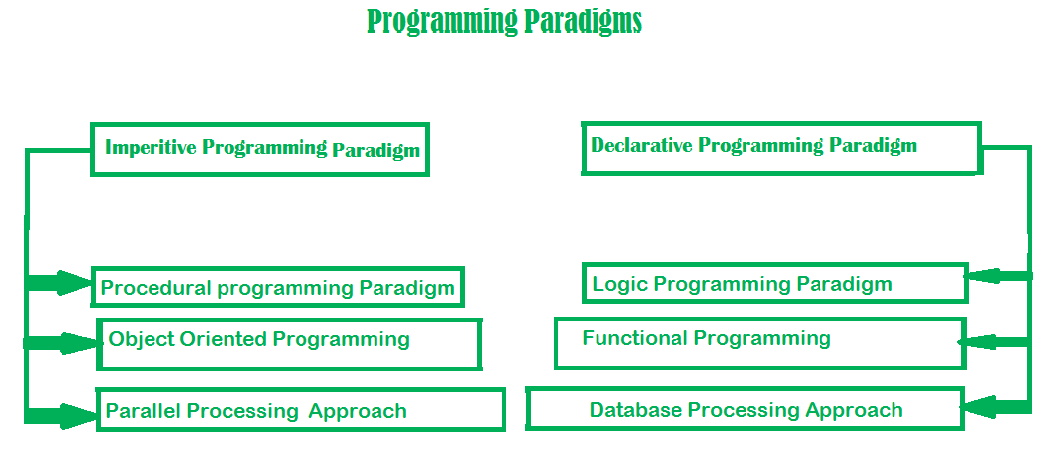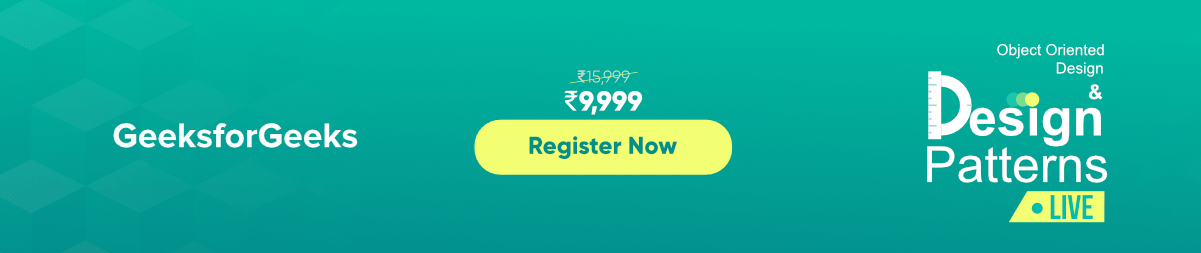Paradigm can also be termed as method to solve some problem or do some task. Programming paradigm is an approach to solve problem using some programming language or also we can say it is a method to solve a problem using tools and techniques that are available to us following some approach. There are lots for programming language that are known but all of them need to follow some strategy when they are implemented and this methodology/strategy is paradigms. Apart from varieties of programming language there are lots of paradigms to fulfil each and every demand. They are discussed below:It is one of the oldest programming paradigm. It features close relation relation to machine architecture. It is based on Von Neumann architecture. It works by changing the program state through assignment statements. It performs step by step task by changing state. The main focus is on how to achieve the goal. The paradigm consist of several statements and after execution of all the result is stored.

1. Very simple to implement
2. It contains loops, variables etc.

1. Complex problem cannot be solved
2. Less efficient and less productive
3. Parallel programming is not possible
```Examples of Imperative programming paradigm:

C : developed by Dennis Ritchie and Ken Thompson
Fortan : developed by John Backus for IBM
Basic : developed by John G Kemeny and Thomas E Kurtz ```

 `// average of five number in C ` ` `  `int` `marks = { 12, 32, 45, 13, 19 } ``int` `sum = 0; ` `float` `average = 0.0; ` `for` `(``int` `i = 0; i < 5; i++) { ` `    ``sum = sum + marks[i]; ` `} ` `average = sum / 5; `

Imperative programming is divided into three broad categories: Procedural, OOP and parallel processing. These paradigms are as follows:

This paradigm emphasizes on procedure in terms of under lying machine model. There is no difference in between procedural and imperative approach. It has the ability to reuse the code and it was boon at that time when it was in use because of its reusability.

```Examples of Procedural programming paradigm:

C : developed by Dennis Ritchie and Ken Thompson
C++ : developed by Bjarne Stroustrup
Java : developed by James Gosling at Sun Microsystems
ColdFusion : developed by J J Allaire
Pascal : developed by Niklaus Wirth ```

 `#include ` `using` `namespace` `std; ` `int` `main() ` `{ ` `    ``int` `i, fact = 1, num; ` `    ``cout << ``"Enter any Number: "``; ` `    ``cin >> number; ` `    ``for` `(i = 1; i <= num; i++) { ` `        ``fact = fact * i; ` `    ``} ` `    ``cout << ``"Factorial of "` `<< num << ``" is: "` `<< fact << endl; ` `    ``return` `0; ` `} `

Then comes OOP,

2. Object oriented programming –
The program is written as a collection of classes and object which are meant for communication. The smallest and basic entity is object and all kind of computation is performed on the objects only. More emphasis is on data rather procedure. It can handle almost all kind of real life problems which are today in scenario.

• Data security
• Inheritance
• Code reusability
• Flexible and abstraction is also present
```Examples of Object Oriented programming paradigm:

Simula : first OOP language
Java : developed by James Gosling at Sun Microsystems
C++ : developed by Bjarne Stroustrup
Objective-C : designed by Brad Cox
Visual Basic .NET : developed by Microsoft
Python : developed by Guido van Rossum
Ruby : developed by Yukihiro Matsumoto
Smalltalk : developed by Alan Kay, Dan Ingalls, Adele Goldberg ```

 `import` `java.io.*; ` ` `  `class` `GFG { ` `    ``public` `static` `void` `main(String[] args) ` `    ``{ ` `        ``System.out.println(``"GfG!"``); ` `        ``Signup s1 = ``new` `Signup(); ` `        ``s1.create(``22``, ``"riya"``, ``"riya2@gmail.com"``, ``'F'``, ``89002``); ` `    ``} ` `} ` ` `  `class` `Signup { ` `    ``int` `userid; ` `    ``String name; ` `    ``String emailid; ` `    ``char` `sex; ` `    ``long` `mob; ` ` `  `    ``public` `void` `create(``int` `userid, String name,  ` `                        ``String emailid, ``char` `sex, ``long` `mob) ` `    ``{ ` `        ``System.out.println("Welcome to  ` `                 ``GeeksforGeeks\nLets create your account\n"); ` `        ``this``.userid = ``132``; ` `        ``this``.name = ``"Radha"``; ` `        ``this``.emailid = ``"radha.89@gmail.com"``; ` `        ``this``.sex = ``'F'``; ` `        ``this``.mob = ``900558981``; ` `        ``System.out.println(``"your account has been created"``); ` `    ``} ` `} `

3. Parallel processing approach –
Parallel processing is the processing of program instructions by dividing them among multiple processors. A parallel processing system posses many numbers of processor with the objective of running a program in less time by dividing them. This approach seems to be like divide and conquer. Examples are NESL (one of the oldest one) and C/C++ also supports because of some library function.

It is divided as Logic, Functional, Database. In computer science the declarative programming is a style of building programs that expresses logic of computation without talking about its control flow. It often considers programs as theories of some logic.It may simplify writing parallel programs. The focus is on what needs to be done rather how it should be done basically emphasize on what code code is actually doing. It just declare the result we want rather how it has be produced. This is the only difference between imperative (how to do) and declarative (what to do) programming paradigms. Getting into deeper we would see logic, functional and database.

It can be termed as abstract model of computation. It would solve logical problems like puzzles, series etc. In logic programming we have a knowledge base which we know before and along with the question and knowledge base which is given to machine, it produces result. In normal programming languages, such concept of knowledge base is not available but while using the concept of artificial intelligence, machine learning we have some models like Perception model which is using the same mechanism.
In logical programming the main emphasize is on knowledge base and the problem. The execution of the program is very much like proof of mathematical statement, e.g., Prolog

```sum of two number in prolog:

predicates
sumoftwonumber(integer, integer)
clauses

sum(0, 0).
sum(n, r):-
n1=n-1,
sum(n1, r1),
r=r1+n ```
The functional programming paradigms has its roots in mathematics and it is language independent. The key principal of this paradigms is the execution of series of mathematical functions. The central model for the abstraction is the function which are meant for some specific computation and not the data structure. Data are loosely coupled to functions.The function hide their implementation. Function can be replaced with their values without changing the meaning of the program. Some of the languages like perl, javascript mostly uses this paradigm.

```Examples of Functional programming paradigm:

JavaScript : developed by Brendan Eich
Haskwell : developed by Lennart Augustsson, Dave Barton
Scala : developed by Martin Odersky
Erlang : developed by Joe Armstrong, Robert Virding
Lisp : developed by John Mccarthy
ML : developed by Robin Milner
Clojure : developed by Rich Hickey ```

The next kind of approach is of Database.

3. Database/Data driven programming approach –
This programming methodology is based on data and its movement. Program statements are defined by data rather than hard-coding a series of steps. A database program is the heart of a business information system and provides file creation, data entry, update, query and reporting functions. There are several programming languages that are developed mostly for database application. For example SQL. It is applied to streams of structured data, for filtering, transforming, aggregating (such as computing statistics), or calling other programs. So it has its own wide application.

```CREATE DATABASE databaseAddress;
PersonID int,
LastName varchar(200),
FirstName varchar(200),
City varchar(200),
State varchar(200)
); ```My Personal Notes arrow_drop_upCheck out this Author's contributed articles.

If you like GeeksforGeeks and would like to contribute, you can also write an article using contribute.geeksforgeeks.org or mail your article to contribute@geeksforgeeks.org. See your article appearing on the GeeksforGeeks main page and help other Geeks.

Please Improve this article if you find anything incorrect by clicking on the "Improve Article" button below.

Article Tags :

9

Please write to us at contribute@geeksforgeeks.org to report any issue with the above content.